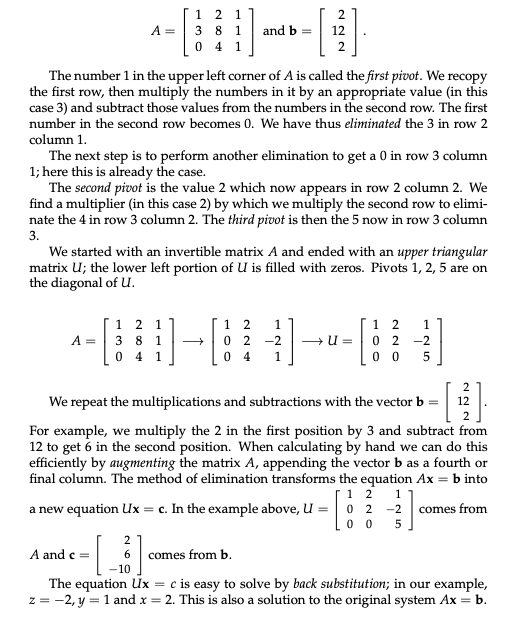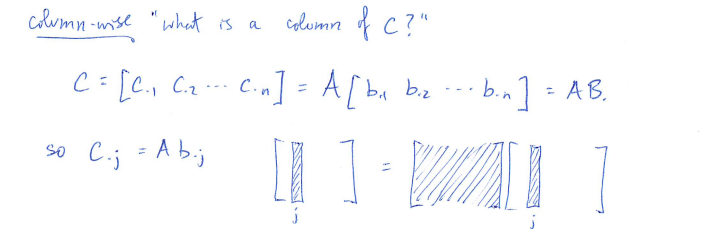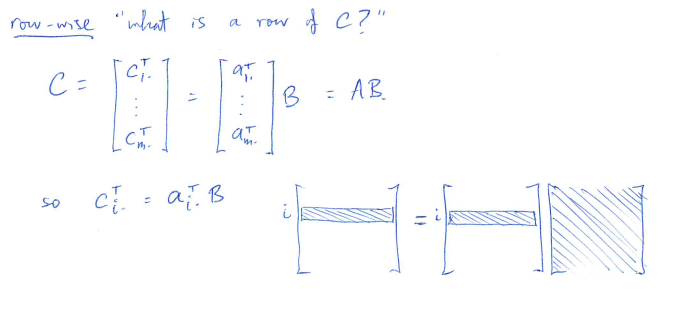### Vaibhav GuptaAI Researcher | Software Engineer

$\newcommand{\mat}{\begin{bmatrix} #1 \end{bmatrix}} \newcommand{\b}{\mathbf{#1}}$

# Linear Systems (Part I)

Essence of Linear Algebra talks about the Matrix as a list of column vectors. In this note, we explore the Matrix as a set of linear equations (row-wise). We go over Gaussian Elimination, Matrix Inversion and A = LU Factorization; the topics that were left out of Essence of Linear Algebra videos. They follow Gilbert Strang’s 18.06 Course on MIT OCW. Also note, for now, we only talk about square matrices and systems where num of linear equations is equal to the number of unknowns.

### Geometry of Linear Equations

The fundamental problem of linear algebra is to solve $n$ linear equations in $n$ unknowns. Below is an example of a linear system of equations (for $n=2$) in matrix form:

\begin{align} A \quad\quad \b{x} \;\; &= \;\; \b{b} \\ \mat{2 & -1\\ -1 & 2} \mat{x \\ y} &= \mat{0 \\ 3}\\ \end{align}

This system of linear equations can be looked at in the following ways:

#### Column-wise (Mixture of Columns)

$A\b{x} = \mat{\b{a_{.1}} & \b{a_{.2}} & \cdots & \b{a_{.n}}} \mat{x_1 \\ x_2 \\ \vdots \\ x_n} = x_1\b{a_{.1}} + x_2\b{a_{.2}} + \cdots + x_n \b{a_{.n}}\\$

where $\b{a_{.i}}$ denotes the $i$-th column vector of matrix $A$.

Geometrically, for our initial example with $n=2$, we want to find number $x$ and $y$ so that $x$ copies of vector $\mat{2 \\ -1}$ added to $y$ copies of vector $\mat{-1 \\ 2}$ equals the vector $\mat{0 \\ 3}$.

#### Row-wise (Dot Product of Rows)

$A\b{x} = \mat{\b{a_{1.}^T} \\ \b{a_{2.}^T} \\ \vdots \\ \b{a_{n.}^T}} \b{x} = \mat{\b{a_{1.}^T}\b{x} \\ \b{a_{2.}^T}\b{x} \\ \vdots \\ \b{a_{n.}^T}\b{x}}$

where $\b{a_{i.}}$ denotes the $i$-th row of matrix $A$. The transpose denotes that it is a row vector and not a column vector. Each $\b{a_{1.}^T}\b{x}$ represents a linear equation in $m$ variables (if $A$ is $n\times m$ matrix).

Geometrically, each such equation represents a $m-1$ dimensional hyperplane in a $m$ dimensional space. Two such equations together represent a $m-2$ dimensional hyperplane, and so on. If $m=3$, then each equation reperesents a plane in $3D$ space and two equations together represent a line in $3D$ space.

### Elimination with Matrices

Elimination is a series of row operations that change your given matrix into an Upper Triangular Matrix (generally denoted by $U$). It is the most common technique used by computers to solve a system of linear equations.

$U = \mat{a & b & c & d\\ & e & f & g \\ & & h & i \\ \huge0 & & &j}$

Allowed Operations for Elimination:

1. Add a multiple of a row to another row.
2. Change the order of rows.

In the equation $A\b{x} = \b{b}$, you have to perform the same row operations on $A$ as you would on $\b{b}$ (to keep the system of equations equivalent). Therefore you can augment $A$ with $\b{b}$ and write it as $\mat{A & \b{b}}$. Once you get $U$, all you need to do is back-substitution.Image from OCW Notes

Pivots may not be $0$. If there is a zero in the pivot position, we must exchange that row with one below to get a non-zero value in the pivot position. If there is a zero in the pivot position and no non-zero value below it, then elimination can not be used to find a unique solution to the system of equations (unique solution doesn’t exist).

How do we represent these elimination steps in matrix form? For this we need to go over matrix-matrix multiplication. We saw this in the light of composition of transformations. Here we explore it further.

### Matrix Multiplication#### Column-wise$C_{.j}$ is a linear combination of the columns of $A$, scaled by the corresponding entries in $\b{b_{.j}}$

#### Row-wise$C_{i.}^T$ is the linear combination of the rows of $B$, scaled according to the corresponding entries in $\b{a^T_{i.}}$. As a result,

\begin{align} \b{a^T_{1.}} = \mat{1 & 0 & \cdots & 0} &\implies C_{1.}^T = B^T_{1.} \\ \b{a^T_{1.}} = \mat{0 & 1 & \cdots & 0} &\implies C_{1.}^T = B^T_{2.} &\textrm{(change order of rows)} \\ \b{a^T_{1.}} = \mat{2 & 3 & \cdots & 0} &\implies C_{1.}^T = 2B^T_{1.} + 3B^T_{2.} &\textrm{(add one row to another)}\\ \end{align}

### Elimination with Matrices (cont.)

From the above discussion, it is evident that we can express elimination operations in matrix form.

$\mat{1 & 0 & 0 \\ -1 & 1 & 0\\ 0 & 0 & 1}\mat{\b{eq1} \\ \b{eq2} \\ \b{eq3}} = \mat{\b{eq1}\\ \b{eq2 - eq1} \\ \b{eq3}}$

The left-most matrix in the above equation is called Elimination Matrix (denoted by $E$). The elimination matrix used to eliminate the entry in row $m$ and column $n$ is denoted $E_{mn}$.

If $A$ is a $3\times 3$ matrix, we can write

$E_{32}E_{31}E_{21}A = U$

Observe that $U$ and $A$ are different matrices (not equivalent). However, the system of linear equations remains equivalent if we apply the same eliminations to both $A$ and $\b{b}$. This is why the method of elimination works. Also observe that the null-space of $U$ and $A$ is the same (substitute $\b{b} = 0$ in the equation $U \b{x} = E \b{b}$).

If one of the pivot elements is coming out to be $0$ and we need to change the order of rows, we use the permutation matrix (denote by $P$). It is obtained by moving around the rows of Identity Matrix $I$. For example,

$P = \mat{0 & 1 & 0 \\ 1 & 0 & 0 \\ 0 & 0 & 1}$

#### Inverse of Elimination Matrix

We have an elimination matrix

$E_{21} = \mat{1 & 0 & 0 \\ -3 & 1 & 0 \\ 0 & 0 & 1}$

which subtracts 3 times row 1 from row 2. To “undo” this operation we must add 3 times row 1 to row 2. This matrix is called the inverse of $E_{21}$ (denoted by​ $E_{21}^{-1}$).

$E_{21}^{-1} = \mat{1 & 0 & 0 \\ 3 & 1 & 0 \\ 0 & 0 & 1}$

In fact, $E_{21}^{-1}E_{21} = I$. Is $E_{21}E_{21}^{-1} = I$ also true? Let us study inverses in more detail.

### Inverse Matrices

The inverse of a square matrix $A$ is another matrix that undoes the translation applied by $A$. It is denoted by $A^{-1}$.

$A^{-1}A = I$

Another property of inverse matrix is $AA^{-1} = I$. According to Gil String, this property is “significantly hard to prove”, but we can get an intuition for it by considering the linear transformations of the matrices. $A$ moves the tip of a vector from point $a$ to point $b$. If $B$ moves the tip of vector from point $b$ to point $a$ , and does it for all the vectors in the vector-space, then $B = A^{-1}$. Now if $B$ is applied to the space first, then $A$ can similarly undo the transformation applied by $B$. Therefore, $A = B^{-1}$ as well.

When would the inverse matrix not exist? If for some $\b{x} \neq \b{0}$, we have $A\b{x} = \b{0}$, the $A^{-1}$ cannot exist. If it existed then $A^{-1}A\b{x} = A^{-1}\b{0} \implies \b{x} = \b{0}$. This makes sense from geometric stand-point as well. $A$ seems to be squishing the space. A vector function (translation is a function), cannot unsquish the space. Thus, if the determinent is zero, then the matrix $A$ is not invertible.

Important Property: $(AB)^{-1} = B^{-1}A^{-1}$

Proof: $(B^{-1}A^{-1})AB = B^{-1}(A^{-1}A)B = B^{-1}IB = B^{-1}B = I$

Finding the inverse of a matrix is closely related to solving systems of linear equations:

$\begin{gather} \mat{1 & 3 \\ 2 & 7} \mat{a & b \\ c & d} = \mat{1 & 0 \\ 0 & 1} \\ (A) \quad (A^{-1}) \quad \quad (I) \\ \end{gather}$

can be read as saying “$A$ times the $j^{th}$ column of $A^{−1}$ equals $j^{th}$ column of the identity matrix”. This is just a special form of the equation $A\b{x} = \b{b}$. Let us see how we can use Gauss Jordan Elimination to solve two or more systems of linear equations at the same time.

#### Gauss Jordan Elimination

We will find the inverse of a matrix using Gauss Jordan Elimination. Just augment the matrix with the whole identity matrix $I$, to obtain $\mat{A \mid I}$.

(Once we have used Gauss’ elimination method to convert the original matrix to upper triangular form, we go on to use Jordan’s idea of eliminating entries in the upper right portion of the matrix.)

We can write the results of the elimination method as the product of a number of elimination matrices $E_{ij}$ with the matrix $A$. Letting $E$ be the product of all the $E_{ij}$, we write the result of this Gauss-Jordan elimination using block matrices:

$E\mat{A|I} = \mat{EA | EI} = \mat{I | E}$

Here $EA = I$, thus $E = A^{-1}$.

Running Time:

1. Forward Triangulation: $O(n^3)$
2. Back Substitution: $O(n^2)$
3. Overall: $O(n^3)$

### A = LU Factorization

We have seen the formula for upper triangular matrix $U = EA$, where $E$ is the composite transformation of various elimination operations (for now assume that the rows of $A$ don’t need any permutation). For example, when $A$ is a $3\times 3$ matrix, $E = E_{32}E_{31}E_{21}$.

If we can find $L = E^{-1}$, then we can write $A = E^{-1}U = LU$. How do we derive $L$? For a $3\times 3$ matrix, $L = E^{-1} = (E_{32}E_{31}E_{21})^{-1} = E_{21}^{-1}E_{31}^{-1}E_{32}^{-1}$. We have already seen how to find the inverse of individual elimination matrices.

The factorization $A = LU$ is preferable to the statement $EA = U$. This is because the row multipliers in $E_{ij}$ directly go into the matrix $L$. On the other hand, $E$ needs to be derived by manually multiplying all the individual $E_{ij}$(s).

We have only talked about the case when no row exchanges are needed. What if we need row exchanges? We multiply the matrix $A$ first with permutation matrices and then with elimination matrices. But how do we find the inverses of permuation matrices? For this we need to talk about Transpose Matrix first.

### Transpose Matrix

We obtain the transpose of a matrix by exchanging its rows and columns. In other words, the entry in row $i$ column $j$ of $A$ is the entry in row $j$ column $i$ of $A^T$. Thus $A^T_{ji} = A_{ij}$

What is $(AB)^T$?

\begin{align} (AB)_{ij} &= A_{i.}B_{.j} \\ \implies (AB)^T_{ji} &= A_{i.}B_{.j} \\ \implies (AB)^T_{ji}&= B^T_{j.}A^T_{.i}\\ \implies (AB)^T&= B^TA^T\\ \end{align}

Also, $(A^T )^{-1} = (A^{-1})^T$

Coming back to inverses of permuation matrices. Below is a permutation matrix that swaps $2$nd row with $3$rd row

$P_{13} = \mat{1&0&0\\0&0&1\\0&1&0} \\$

The inverse of any permutation matrix $P$ is $P^{−1} = P^T$.